# Riesz summation method

(diff) ← Older revision | Latest revision (diff) | Newer revision → (diff)

A method for summing series of numbers and functions; denoted by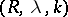. A seriesis summable by the Riesz summation methodto the sumif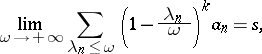where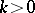,, andis a continuous parameter. The method was introduced by M. Riesz  for the summation of Dirichlet series. The methodis regular; whenit is equivalent to the Cesàro summation method(cf. Cesàro summation methods), and these methods are compatible (cf. Compatibility of summation methods).

Riesz considered also a method in which summability of the series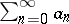is defined by means of the limit of the sequence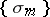, where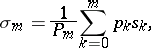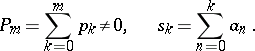This method is denoted by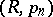. The methodis a modification of the method(when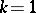) and is a generalization of it to the case of an arbitrary.

How to Cite This Entry:
Riesz summation method. Encyclopedia of Mathematics. URL: http://encyclopediaofmath.org/index.php?title=Riesz_summation_method&oldid=19011
This article was adapted from an original article by I.I. Volkov (originator), which appeared in Encyclopedia of Mathematics - ISBN 1402006098. See original article